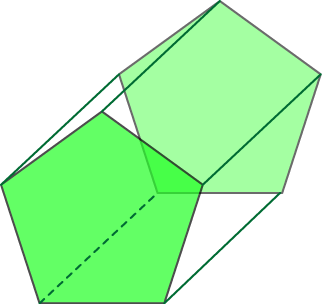# Definition of PrismA prism is a three-dimensional object, both ends of which are identical.

Every cross-section of a prism has the same shape as the ends.

Prisms are one form of polyhedron.

Each side of a prism is a parallelogram. If the two ends are exactly over each other, then the sides are rectangles and we call the prism a "right prism".

The shape of the base of a prism gives the solid its name. For example, if the base is a pentagon, then the prism is called a pentagonal prism.

### Description

The aim of this dictionary is to provide definitions to common mathematical terms. Students learn a new math skill every week at school, sometimes just before they start a new skill, if they want to look at what a specific term means, this is where this dictionary will become handy and a go-to guide for a student.

### Audience

Year 1 to Year 12 students

### Learning Objectives

Learn common math terms starting with letter P

Author: Subject Coach
You must be logged in as Student to ask a Question.